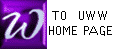INTEGRATION BY SUBSTITUTION
Prof McFarland seeks comment on this page.

 Since the Chain rule tells us dydx = dydu . dudx , we may anti-differentiate both sides to get:
 y(x) =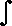dydu . dudx dx . To use this tool, we must first choose a "u": see METHODS.


 In finding3x ( x2 + 8 )8dx , mark the correct "u" and copy it to the answer box below.
Use left mouse button to highlight u =


 In finding(3x2 - 1 ) ex3 - xdx , mark the correct "u" and copy it to the answer box below.
You choose u =


In finding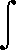2x4x5 + 1
dx , mark the correct "u" and copy it to the answer box below.
You choose u =


 In finding1    x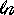x dx   , mark the correct "u" and copy it to the answer box below.
You choose u =


 In finding( x + 1 )( x - 2 )9 dx , mark the correct "u" and copy it to the answer box below.
You choose u =

 Could you solve this problem using Integration by Parts?


In finding4x3 + 6x2 - 1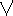x4 + 3x2 - x
dx,   mark the correct "u"; copy it to the answer box below.
You choose u =


 In finding( x + 1 ) dx , mark the correct "u" and copy it to the answer box below.
You choose u =